# Legendre polynomials

(Redirected from Legendre polynomial)

In mathematics, the Legendre polynomials Pn(x) are orthogonal polynomials in the variable −1 ≤ x ≤ 1. Their orthogonality is with unit weight,$\int_{-1}^{1} P_{n}(x) P_{n'}(x) dx = 0\quad \hbox{for}\quad n\ne n'.$

In physics they commonly appear as a function of a polar angle 0 ≤ θ ≤ π with x = cosθ$\int^{\pi}_{0} P_{n}(\cos\theta) P_{n'}(\cos\theta) \sin\theta \;d\theta = 0\quad \hbox{for}\quad n\ne n'.$.

The polynomials as function of cosθ are part of the solution of the Laplace equation in spherical polar coordinates.

By the sequential Gram-Schmidt orthogonalization procedure applied to {1, x, x², x³, …} the nth degree polynomial Pn can be constructed recursively. The Gram-Schmidt procedure applies to all members of the family of orthogonal polynomials, such as Hermite polynomials, Chebyshev polynomials, etc. Further, Pn(x) has in common with the other orthogonal polynomials that it has exactly n real distinct zeroes. These zeroes are used as grid points in Gauss quadrature (numerical integration) schemes.

## Historical note

The polynomials were named "Legendre coefficients" by the British mathematician Isaac Todhunter in honor of the French mathematician Adrien-Marie Legendre (1752–1833), who was the first to introduce and study them. Todhunter called the functions "coefficients", instead of "polynomials", because they appear as coefficients of hn in the expansion of the generating function (see below); Todhunter also introduced the notation Pn, which is still generally used.

Legendre's polynomials have been introduced by Legendre in a memoir Sur l'attraction des sphéroïdes homogènes published in the Mémoires de Mathématiques et de Physique, présentés à l'Académie royale des sciences par sçavants étrangers, Tome x, pp. 411–435, Paris, 1785.. The functions also occur in a memoir by Laplace received by the Academy in 1782, Théorie des attractions des sphéroïdes et de la figure des planètes, but the original introduction of the functions appears nevertheless to be due to Legendre, whose work was not published for several years after it was written; his memoir is mentioned as approved for publication in the report of the sittings of the Academy for 1783. Legendre himself declares that Laplace introduced the potential (i.e., generating) function, but that he himself developed the expansion. Later, the mathematicians Jacobi, Dirichlet, and Heine have written on the question of priority and agree that Legendre deserves the credit.

## Rodrigues' formula

The French amateur mathematician Olinde Rodrigues (1795–1851) proved the following formula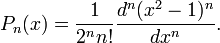$P_n(x) = {1 \over 2^n n!} \frac{d^n(x^2 -1)^n}{dx^n} .$

Using the Newton binomial and the equation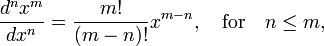$\frac{d^n x^m}{dx^n} = \frac{m!}{(m-n)!} x^{m-n}, \quad\hbox{for}\quad n\le m,$

we get the explicit expression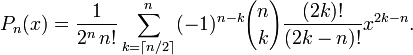$P_n(x) = \frac{1}{2^n \, n!}\sum_{k=\lceil n/2 \rceil}^n (-1)^{n-k} {n \choose k}\frac{(2k)!}{(2k-n)!} x ^{2k-n} .$

Substitution p=n-k gives this formula a slightly different appearance$P_n(x) = \frac{1}{2^n} \sum_{p=0}^{\lfloor n/2 \rfloor} (-1)^p \frac{(2n-2p)!}{p!(n-p)!(n-2p)!} x^{n-2p}.$

## Generating function

The coefficients of hn in the following expansion of the generating function are Legendre polynomials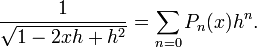$\frac{1}{\sqrt{1-2xh+h^2}} = \sum_{n=0} P_n(x) h^n .$

The expansion converges for |h| < 1. This expansion is useful in expanding the inverse distance between two points r and R$\frac{1}{|\mathbf{r}-\mathbf{R}|} = \frac{1}{\sqrt{r^2 + R^2 -2rR\cos\gamma}}= \frac{1}{R} \frac{1}{\sqrt{h^2 + 1 -2hx}},$

where$h\equiv \frac{r}{R}\quad \hbox{and}\quad x \equiv \cos\gamma \equiv \mathbf{r}\cdot\mathbf{R}/(rR).$

Obviously the expansion makes sense only if R > r. The function appears in Newton's gravitational potential and in Coulomb's electrostatic potential.

## Normalization

The polynomials are not normalized to unity, but$\int_{-1}^{1} P_{n}(x) P_{m}(x) dx = \frac{2}{2n+1} \delta_{n m},$

where δnm is the Kronecker delta.

## Differential equation

The Legendre polynomials are solutions of the Legendre differential equation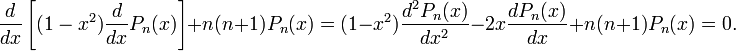$\frac{d}{dx} \left[ (1-x^2) \frac{d}{dx} P_n(x) \right] + n(n+1)P_n(x) = (1-x^2) \frac{d^2 P_n(x)}{dx^2} - 2 x \frac{dP_n(x)}{dx} + n(n+1) P_n(x) = 0.$

This differential equation has another class of solutions: Legendre functions of the second kind Qn(x), which are infinite series in 1/x. These functions are of lesser importance.

Note that the differential equation has the form of an eigenvalue equation with eigenvalue −n(n+1) of the operator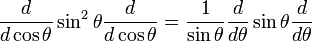$\frac{d}{d\cos\theta} \sin^2\theta \frac{d}{d\cos\theta} = \frac{1}{\sin\theta} \frac{d}{d\theta} \sin\theta \frac{d}{d\theta}$

This operator is the θ-dependent part of the Laplace operator ∇² in spherical polar coordinates.

## Properties of Legendre polynomials

Legendre polynomials have parity (−1)n under x → -x,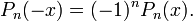$P_n(-x) = (-1)^n P_n(x). \,$

The following condition normalizes the polynomials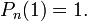$P_n(1) = 1. \,$

## Recurrence Relations

Legendre polynomials satisfy the recurrence relations$(1-x^2) \frac{d}{dx} P_n = (n+1) xP_n - (n+1) P_{n+1} = n P_{n-1} - nx P_n$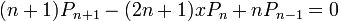$(n+1) P_{n+1} - (2n+1) x P_n + n P_{n-1} = 0 \,$

From these two relations follows easily$\frac{d \big(P_{n+1} - P_{n-1}\big)}{dx} = (2n+1) P_n .$

Weisstein, Eric W. "Legendre Polynomial." From MathWorld--A Wolfram Web Resource. 

## Footnote

1. I. Todhunter, An Elementary Treatise on Laplace's, Lamé's, and Bessel's Functions, MacMillan, 1875 (London) Google book.
2. Full text online: Recherches Sur l'attraction des sphéroïdes homogènes par M. Le Gendre. Retrieved September 10, 2009.